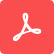# Grid Specification

By Emerson Melo, Tom Chen and Xin Lei

In this tutorial, you will learn how to set up the FDTD grid to obtain accurate simulation results. We will demonstrate the differences between uniform and non-uniform grids, and how to utilize them to optimize the simulation mesh. Additionally, you will learn how to calculate the symmetric and anti-symmetric modes of a directional coupler.

Share On:
Additional information: This Tutorial was updated in Aug 31, 2023Tidy3D GUI: Tutorial 7

In this tutorial, we'll use the directional coupler example from the FDTD examples folder to explore the "Grid Specification" options. The coupling coefficient (C) and the cross-over length (L) are two critical quantities for designing and analyzing such a device.

Both quantities depend on the difference between the effective indices of the symmetric and antisymmetric modes of the coupled waveguides, denoted here as n0 and n1, respectively. Let's set up the ModeSolver to calculate these eigenmodes and illustrate the effects of different grid meshes.

Start by including a "ModeMonitor" positioned at the center of the coupling region, with dimensions of 3 microns in the y-direction and 1.1 microns in the z-direction. Then, set its parameters as shown here. Click "Add ModeSolver" and configure the newly created mode solver to calculate the first two modes within this plane. You can refer to the previous tutorials to accomplish this task if needed.

Click on "Grid Specification" and select the "UniformGrid" type. Set the "dl" parameter, which controls the grid size, to 50 nanometers. Choose the 2D Chart visualization, and leave enabled only the "Show grid" checkbox. As you can see, the simulation domain consists of an evenly-spaced grid mesh. Select the mode solver and run the simulation. After the mode calculation, you'll observe the electric field distribution of the first mode. If you select the "Ey" field component, you'll recognize it as the symmetric mode. To visualize the antisymmetric one, set the Mode Index to 1. It's worth noting that the grid size limits the image resolution. Now, switch to the Effective Index visualization to obtain the information needed for calculating the coupling coefficient and the cross-over length.

In some cases, you may reduce the grid size to enhance simulation accuracy. To illustrate this, click the "Edit" button to create a new task version, and change the "dl" value to 20 nanometers. You'll notice a thinner yet evenly spaced mesh in the 2D Chart view. After running the mode solver, you'll see improved images of the field distributions due to the higher mesh resolution. In this case, the effective indices are 2.473 and 2.438 for the symmetric and antisymmetric optical modes, respectively, resulting in an effective index difference of 0.035. However, the total number of grid points in the computational domain increased from 4 to 50 million, leading to higher computational costs. Sometimes, a non-uniform mesh can balance cost and accuracy better.

So, create a new task version and click "Grid Specification" again. This time, choose the "AutoGrid" mesh type. Increase the "minimum steps per wavelength" slightly, from 10 (the minimum recommended value) to 15, and set the "wavelength" to 1.55. This information is used to automatically calculate different grid sizes for each medium, resulting in a denser mesh on the silicon regions, with a grid spacing of over 29 nm, and a coarser mesh on the lower refractive index region, including the space between the waveguides. Run the mode solver again and review the results. With the non-uniform mesh, you can still observe the details of the field distributions for both optical modes. The effective indices are now 2.463 and 2.429, and the simulation domain has 7 million grid points.

Lastly, let's refine the mesh between the waveguides. Click the "Edit" button and select "Grid Specification" again. Include an "Add Override Structure," choose a "Box" geometry type, and set its dimensions to 10 microns in the x-direction, 0.4 microns in the y-direction, and 0.3 microns in the z-direction. Next, set the grid size to 20 nanometers in all directions. After confirming the changes, you'll see a grid override structure between the directional coupler waveguides. In the 2D Chart view, you can clearly see the uniform and thinner mesh within the green rectangle. Start the mode solver again and go to the post-run results. The resulting electric field distribution is as detailed as in the thinner uniform mesh case, but the simulation domain has only 10 million elements. In this last case, the mode effective indices are 2.466 and 2.433, resulting in an effective index difference of 0.033.

You can fully customize the simulation grid by unchecking the "Use the same grid spec for all directions" checkbox, allowing you to define different grid types for the x, y, and z directions.